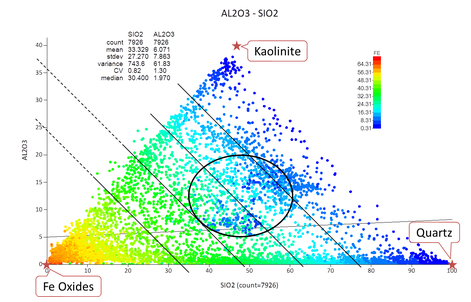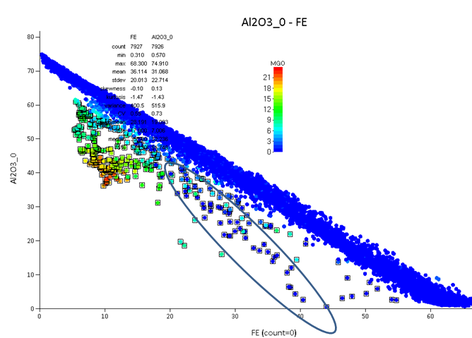# Exploratory Data Analysis in Practice: Iron Ore Geochemistry and Mineralogy

I recently have had the pleasure to perform some beta testing of a novel software, X10-Geo, by Phinar Software. As I was performing this testing I made notes to provide feedback to Rob de Bruin, who is behind Phinar. By the end I looked at these raw notes and thought the work would make a nice example illustrating the step by step digging through data during exploratory data analysis (EDA) and, I decided to write up the results as a little case study. So here it is.

The main geochemistry of the common BIF-hosted Iron Ore deposits can be described as a mixing system of Fe-oxides, Quartz, Kaolinite. The scatter plot of Al2O3 versus SiO2 in Figure 1 illustrates iron ore samples relative to key constituent minerals and mixing/enrichment trends.Figure 1: Al2O3 - SiO2 scatter plot with the Iron Ore key constituent minerals, Fe-oxides - Kaolinite - Quartz.

While the genesis of Iron Ore deposits continues to be debated, Quartz is a mobile mineral in the system and its relative depletion converts primary BIF into iron ore (“enrichment”). Kaolinite in these deposits is derived from shale bands and is geochemically immobile. In samples the shale bands get mixed with the Fe/SiO2 rich layers, see “mixing” trend in Figure 1 for enriched iron ore mixing with Kaolinite. Note that the “enrichment” trend can also get realized via the mixing of strongly enriched rocks with partially or non-enriched rocks.

### Mineralogy model for Iron Ore Assays

Starting with this general model of the iron ore geochemistry it is possible to drill down into further particularities of a given data set. First screening of the data points show some “outlier” samples above the “mixing” line in Figure 1. These highlight the simplification of the model; the observed “excess” Al2O3 is caused by two factors firstly, the Al-mineral Gibbsite is known to occur in parts of these deposits and, secondly, the Fe-minerals show replacement of Fe(3+) by Al(3+).Figure 2 Al2O3 - SiO2 scatter plot coloured by Fe grade showing a set of iso-Fe lines.

In Figure 2, the Al2O3 - SiO2 scatter plot is coloured by Fe grade which results in a regular “striping” apart from some “outlier” points in the central area of the plot (black ellipse). To gain a better understanding of those outliers in the data set it is desirable to isolate the “well-behaved” samples, which appear to adhere to the three-minerals mixing system introduced above.

The “well-behaved” samples can be characterized by constant Fe while Kaolinite and Quartz vary systematically. This variation follows the parallel lines in Figure 2, representing a set of Fe-isolines, which can be described with a linear function;

with the gradient m and  the intercept of the function with the y-axis. The gradient m can be calculated using the mass percentage differences of and  between Quartz and Kaolinite:

Using -3/4  as approximation for gradient m, Equation (1) can be solved for Al2O3(0) and gives an expression that captures the “striping” effect observed in Figure 2:

The equation (2) can be implemented in the data set to make it available as a derived variable. Figure 3 shows the implementation in the Data Loader of X10 Geo.

## Testing the Mineralogy Model

In the next step the explanatory power of this derived variable, Al2O3(0), is analysed using its scatter plot with Fe, see Figure 4. The graph on the right in Figure 4 demonstrates that the previously observed outliers have been successfully delineated.Figure 4 Highlighting outliers of the three-minerals system using derived variable Al2O3(0)

As this little case study was compiled as part of beta testing for X10 geo the full capabilities were not understood. When I got to this point in twisting the data, I discovered the really powerful generic implementation of the 3D plot utility in X10 Geo. Primarily the axes defaults are geographic coordinates however, they can be set to any variable available in the data set. This way I could generate 3D plot shown in Figure 5 which I rotated such that the outliers delineated above stand out again.

How easy! And clearly, the data points that adhere to the three-minerals system sit close to a plane in this 3D space, while the outliers are some distance away from the plane. Note also that the variable reduction performed above through introducing the derived variable Al2O3(0) is equivalent to a projection of the data points in the 3D plot onto a plane with normal vector in Equation (2).

So the flexible 3D plotting feature facilitates the data analysis considerably yet, using variable Al2O3(0) is still useful when looking for further detail behind those outliers. Figure 6  displays the scatter plot Al2O3_0 – Fe, coloured by MgO concentration.  It can be seen that most outliers are related to a MgO component, likely to be a carbonate. The data points in the blue ellipse belong to yet another rock type (high sulphur).Figure 6 Al2O3(0) - Fe scatter plot coloured by MgO concentration.

In conclusion most samples in the data adhere to a simple mixing system between FE, Al2O3 and SiO2 plus other correlated elements (likely LOI), which have not been investigated here. On a mineralogy basis the system consists of {Fe-oxide, Kaolinite, Quartz}. Starting with this kind of model hypothesis gives clear guidance to the EDA process, which can enhance focus on parts of the data set that are not explained by the hypothesis and require other ideas and their testing.

When this type of data analysis is done with a spatial data set further analysis is required to investigate the potential spatial coherence of any identified population. Where a coherent and geologically reasonable region can be identified, it should be used as spatial domain which can greatly improve the quality of generated deposit model.